# Inverse Functions Worksheet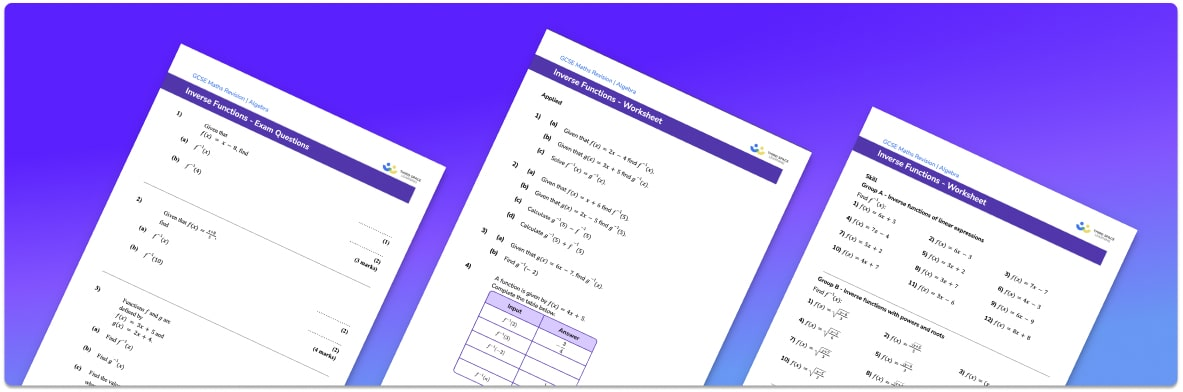• Section 1 of the inverse functions worksheet contains 20+ skills-based inverse functions questions, in 3 groups to support differentiation
• Section 2 contains 4 applied inverse functions questions with a mix of worded problems and deeper problem solving questions
• Section 3 contains 3 foundation and higher level GCSE exam questions on inverse functions
• Answers and a mark scheme for all inverse functions questions are provided
• Questions follow variation theory with plenty of opportunities for students to work independently at their own level
• All questions created by fully qualified expert secondary maths teachers
• Suitable for GCSE and IGCSE maths revision for AQA, OCR and Edexcel exam boards

• This field is for validation purposes and should be left unchanged.

You can unsubscribe at any time (each email we send will contain an easy way to unsubscribe). To find out more about how we use your data, see our privacy policy.

### Inverse functions at a glance

The inverse of a function f is the reverse of the function; it is denoted f-1 and it takes us back to the original value, before that function was applied. For example, taking the square root of a number is the inverse of squaring a number.

We can find the inverse of linear functions, functions involving powers and trigonometric functions. The inverse of a function can be used to solve an equation or find a missing value on a graph. Inverse trigonometric functions are used when finding missing angles in triangles.

To find the inverse of a composite function we first rewrite the equation, replacing any x with a y and setting the equation equal to x. We then rearrange the equation to make y the subject. Finally we replace y with f-1(x).

Looking forward, students can progress with more functions worksheets, to additional algebra worksheets, for example a solving equations worksheet orFor more teaching and learning support on algebra our GCSE maths lessons provide step by step support for all GCSE maths concepts.

## Related worksheets

Composite and Inverse Functions Worksheet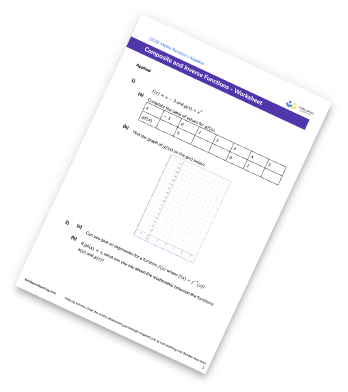Function Notation Worksheet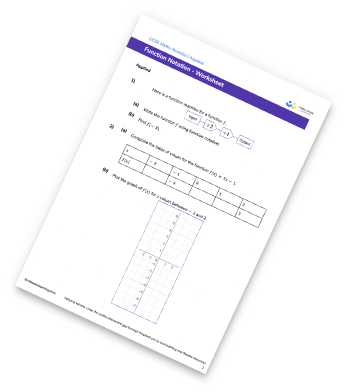Function Machines Worksheet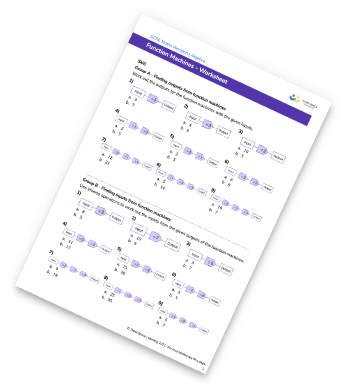Functions Worksheet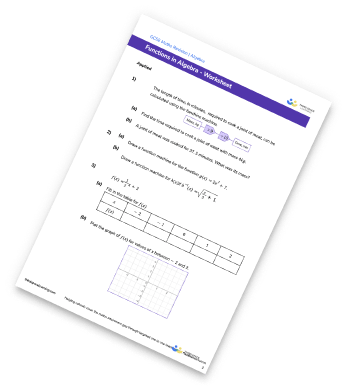## Do you have KS4 students who need more focused attention to succeed at GCSE?There will be students in your class who require individual attention to help them succeed in their maths GCSEs. In a class of 30, it’s not always easy to provide.

Help your students feel confident with exam-style questions and the strategies they’ll need to answer them correctly with our dedicated GCSE maths revision programme.

Lessons are selected to provide support where each student needs it most, and specially-trained GCSE maths tutors adapt the pitch and pace of each lesson. This ensures a personalised revision programme that raises grades and boosts confidence.

Find out more